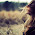# Find out the repeated or duplicate element in an array in Java

An interviewer asked me this question in an interview. There could be many ways, you can check if your array contains duplicate elements or not. If you gave answer to this question and interviewer wants to test your programming skills then interviewer may ask about the time-space complexity about your algorithm and also say to find out another way if your algorithm is not an optimized one.

### Ways to check Array for duplicate elements Java

In this tutorial, we will see couple of ways to find out the duplicate or repeated elements in an array. Some of the ways are given below:

#### Solution 1

```public void findDuplicate(int arr[])
{
System.out.println("The repeating elements are: ");
for (int index = 0; index < arr.length; index++)
{
if (arr[Math.abs(arr[index])] >= 0)
arr[Math.abs(arr[index])] = -arr[Math.abs(arr[index])];
else {
System.out.println(Math.abs(arr[index]));
}
}
}
```

#### Solution 2

```public void findDuplicate(int[] arr) {
for (int index = 0; index < arr.length; index++) {
for (int index1 = 0; index1 < arr.length; index1++) {
if (arr[index] == arr[index1] && index != index1) {
System.out.println(arr[index]);
}
}
}
}
```

#### Solution 3 (Using Set)

```public void findDuplicate(Integer[] arr) {
Set hashSet = new HashSet();
for (Integer num : arr) {
System.out.println(num);
}
}
}
```

#### Solution 4 (Using List)

```public int findDuplicate(List<Integer> arr){
int length = arr.size() - 1;
int total = getTotal(numbers);
int duplicate = total - (length*(length + 1)/2);
return duplicate;
}

public int getTotal(List<Integer> arr){
int sum = 0;
for (int num : arr) {
sum += num;
}
return sum;
}
```

I tried my best to provide possible solutions to find out the duplicate elements in an array. Please provide your valuable comments, suggestions and feedback to correct any mistakes made or to make content valuable for other users.

1.Solution 2 takes more than required iterations, you can actually reduce the outer loop iterations.

2.Could you please explain how first method is working?

3.time complexity of second solution is O(n^2), and for the third solution is O(n).